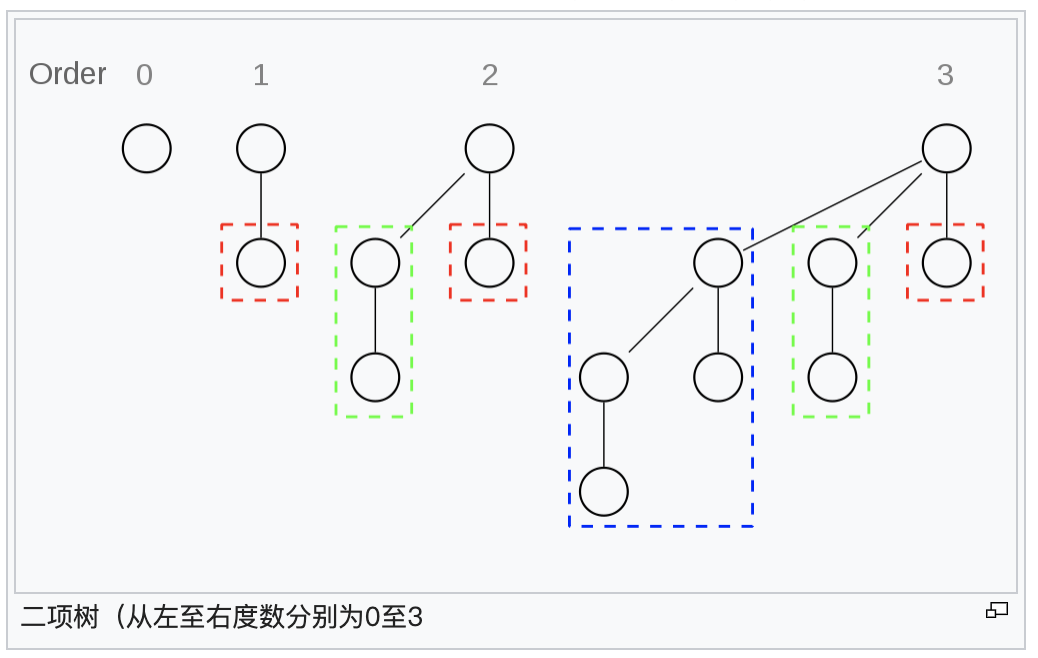heap代码

binary heap代码

# binomial heap

## 堆的具体形状

*** 图片源于wiki***## push

$f(x)>=1$的时候$x%2=1$,
$f(x)>=2$的时候$x%4=3$,
$f(x)>=3$的时候$x%8=7$

\begin{aligned} \sum_{i=1}^n{f(i)}=\lfloor\frac{x+1}{2}\rfloor+\lfloor\frac{x+1}{4}\rfloor+\lfloor\frac{x+1}{8}\rfloor+…+\lt x+1 \end{aligned}

binary heap代码

# fibonacci heap

## top

$O(1)$

## D(H)

### 斐波拉契数列

\begin{aligned} F_i = \left{ \begin{aligned} &0&i=0\ &1&i=1\ &F_{i-2}+F_{i-1]}&i\ge 2\ \end{aligned} \right. \end{aligned}

### 斐波拉契数列的两个结论

$F_{n+2}=1+\sum_{i=1}^nF_i$
$F_{n+2}\ge \phi^n,\phi^2=\phi+1,\phi大约取1.618$

fibonacci heap代码

# pairing heap

## potential method

$F(H) = \sum F(node)$,
$F(node_i)=1-min(d_i,\sqrt{n})$

pairing heap代码

# leftist heap

## merge

$O(lgn)$, 当我们合并两个堆$H1,H2$的时候，我们只需要比较这两个堆顶的大小，不妨设H1小，并设H3、H4为H1的左右儿子，则我们可以这样来看待，我们将H3,H4从H1中断开，递归合并H4和H2位H5，这时候我们还剩下H3、H5以及H1的堆顶，我们根据左偏树的定义，选择H3、H5分别作为左右或右左儿子即可，

leftist heap代码

skew heap代码